• github主页 https://github.com/tidyverse/ggplot2 ggplot2中的函数：https://ggplot2.tidyverse.org/reference/index.html 图形范例：...install.packages("ggplot2") 使用 librar...

github主页
https://github.com/tidyverse/ggplot2
ggplot2中的函数：https://ggplot2.tidyverse.org/reference/index.html
图形范例：http://www.ggplot2-exts.org/gallery/
安装

install.packages(
"ggplot2")

使用
library("ggplot2")

ggplot(data = <DATA>) + <GEOM_FUNCTION>(mapping = aes(<MAPPINGS>))

ggplot(data = <DATA>) + <GEOM_FUNCTION>(mapping = aes(<MAPPINGS>), stat = <STAT>, position = <POSITION>) + <COORDINATE_FUNCTION> + <FACET_FUNCTION>

ggplot2绘图中的基本概念
ggplot图形系统的核心理念是把绘图和数据分离，吧数据相关的绘图与数据无关的绘图分离，按照图层做图。ggplot2可以把绘图拆分成多个图层，且能够按照顺序创建多重图形。
使用ggplot2包创建图形时，每个图形都是由函数 ggplot() 创建的，提供绘图默认的数据和映射关系，其他图层中可以继承该映射关系或者修改该映射关系。

ggplot(data = NULL, mapping = aes())

数据通过参数 data 指定：数据框对象
映射通过参数 mapping 指定：有函数 aes() 来设置映射
由几何对象来控制绘制的几何图形，通过 + 来增加图形的元素，这使得绘制图形的过程结构化，同时使绘图更具灵活性。
在 ggplot2 中，图形语法中至少包含如下几个图形部件，每一个部件可以是一个图层，这些组件之间以图层的方式来粘合构图。每个图层可以代表一个图形组件，这些图形组件以图层的方式叠加在一起构成一个绘图的整体，在每个图层中的图形组件又可以分别设定数据、映射或其他相关参数，因此这些组件之间又是具有相对独立性的。
几何对象 geom_XXX
统计变换  stats
标度 scale
坐标系 coord
分面 facet
主题 theme
1. 映射
映射即为数据集中的数据关联到相应的图形属性过程中的一种对应关系，是将一个变量中离散或者连续的数据与一个图形属性中以不同的参数来相互关联，而设定能够将这个变量中所有的数据统一为一个图形属性。aes()函数是ggplot2中的映射函数。
aes() 函数中常见的映射选项是：
x y ：用于指定x轴和y轴映射的变量
color：映射点或线的颜色
fill：映射填充区域的颜色
linestyle：映射图形的形状
size：点的尺寸和线的宽度
shape：映射点的形状
group：默认情况下 ggplot2 把所有的观测值分为了一组，如果需要把观测值按额外的离散变量进行分组处理，必须修改默认的分组设置
映射和设定是不同的
映射是将一个变量中离散或连续的数据与一个图形属性中以不同的参数来相互关联
设定能够将这个变量的所有数据统一为一个图形的属性

p <- ggplot(data = mtcars, mapping = aes(wt, mpg))
p + geom_point(color = "blue")   # 设定散点的颜色为蓝色
p + geom_point(aes(color = "blue"))   # 错误的映射，在 aes() 函数中 color = "blue" 的实际意思是将“blue”当成一个变量，用这个变量里的数据去关联图形属性中的参数，因为“blue”只含有一个字符变量，默认情况下为离散变量，按默认的颜色标度标记为桃红色。

2. 分组
默认情况下 ggplot2 把所有观测点分为一组，如果需要把观测点按照额外的离散变量进行分组处理，必须修改默认的分组设置。

p1 <- ggplot(data = mtcars, mapping = aes(x = wt, y = hp)) + geom_point() + geom_line()  # 默认分组设置，即 group = 1
p2 <- ggplot(data = mtcars, mapping = aes(x = wt, y = hp, group = factor(gear), color = factor(gear))) + geom_point() + geom_line()  # 把wt和hp所对应的的观测点按照gear（gear以因子变为离散变量）进行分组

3. 图层
每个图层可以代表一个图形组件，例如下面要介绍的几何对象、统计变换等图形组件，这些组件以图层的方式叠加在一起构成一个绘图的整体，在每个图层中的图形组件又可以分别设定数据、映射或其他相关参数，因此组件之间是具有相对独立性的。图层之间有 + 连接
3.1 在几何图形中设置映射

p <- ggplot(mtcars, aes(x = mpg, y = wt, color = factor(gear)))   # 设定默认的映射关系
p + geom_point()  # 沿用默认的映射关系来绘制散点图
p + geom_point(aes(shape = factor(carb)))  # 添加图层中的shape的映射关系
p + geom_point(aes(y = carb))  # 修改默认的y的映射关系，注意图中y轴名称仍然以默认的wt表示
p + geom_point(aes(color = NULL))  # 删除默认的color映射关系

3.2 采用多个数据集或向量数据绘图

mtcars.c <- transform(mtcars, mpg = mpg^2)  # 构建不同于mtcars的数据集mtcars.c
ggplot() +
geom_point(data = mtcars, aes(x = hp, y = mpg), data = mtcars, color = "red") +
geom_point(aes(x = mtcars$hp, y = mtcars$disp), color = "green") +   # 选用向量数据
geom_point(data = mtcars.c, aes(x = hp, y = mpg), color = "blue") +   # 选用不同的数据集
geom_line(data = mtcars.c, aes(x = hp, y = mpg), color = "blue")

4. 几何对象和统计变换
几何对象执行着图层的实际渲染，控制着生成的图形类型。例如用 geom_point() 将会生成散点图，geom_line() 将会生成折线图，
统计变换即对数据进行统计变化，通常以某种方式对数据信息进行汇总，例如通过 stat_smooth() 添加光滑曲线
geom_point()绘图

p <- ggplot(mtcars, aes(x = wt, y = mpg)
p + geom_point()
p + geom_point(aes(color = qsec))    # 更改颜色 连续变量
p + geom_point(aes(color = factor(gear)))   # 更改颜色 离散变量
p + geom_point(aes(alpha = qsec))   # 更改透明度
p + geom_point(aes(shape = factor(gear)))   # 更改形状
p + geom_point(aes(size = qsec))   # 更改点大小
p + geom_point(color = "grey50", size = 5) + geom_point(aes(color = qsec), size = 4)   # 两种颜色叠加
p + geom_point(color = "grey50", size = 5) + geom_point(aes(shape = factor(gear)), size = 3)  # 颜色和形状叠加

geom_histogram() 绘图 该几何形状默认使用 stat_bin 这个统计变换，而这个统计变换会生成 count, density, x 三个变量。 count：每个组里观测值的数目  density：每个组里观测值的密度  x：组的中心。生成的变量在ggplot()中
geom_smooth() 用来给数据添加平滑曲线，所能采用的方法包括 lm glm gam loess rlm 等， 这些方法需要通过加载公式来实现。
5 分面（facet）
即在一个页面上自动摆放多幅图形，这一过程先将数据划分为多个子集，然后将每个子集依次绘制到页面的不同面板中。
ggplot2提供了两种分面类型：
网格型（facet_grid）：生成的是一个2维的面板网格，面板的行与列通过变量来定义，本质是2维的。
封面型（facet_wrap）：生成一个1维的面板条块，然后再分装到2维中，本质是1维的。
6 标度 （scale）
标度控制着数据到图形属性的映射，更重要的一点是标度将我们的数据转化为视觉上可以感知的东西，如大小、颜色、位置、形状等，所以可以通过标度修改坐标轴和图例的参数。
常用的标度有：标签 图形选项 坐标轴
7. 坐标系

8. 主题（theme）
主题系列控制着图形中的非数据元素外观，它不会影响几何对象和标度等数据元素。主题修改是一个对绘图精雕细琢的过程，主要对标题、坐标轴标签、图例标签等文字调整，以及网格线、背景、轴须的颜色搭配。

保存图片
方式一 使用 R 中的方式
pdf(file = "picture.pdf")
ggplot(mtcars, aes(x = wt, y = mpg)) + geom_point()
dev.off()

png(file = "myplot.png", width = 400, height = 400)
ggplot(matcars, aes(x = wt, y = mpg)) + geom_point()
dev.off()
方式二 使用 ggsave() 函数
ggsave(p, filename = "p.pdf", width = 12, height = 9)
ggsave(p, filename = "p.png", width = 12, height = 9)
使用 ggplot2 自带的数据集 mpg

ggplot(data = mpg) + geom_point(mapping = aes(x = displ, y = hwy))

ggplot(data = mpg) + geom_point(mapping = aes(x = displ, y = hwy, color = class))

ggplot(data = mpg) + geom_point(mapping = aes(x = displ, y = hwy, size = class))

ggplot(data = mpg) + geom_point(mapping = aes(x = displ, y = hwy, alpha = class))    # alpha 指透明度

ggplot(data = mpg) + geom_point(mapping = aes(x = displ, y = hwy, shape = class))

ggplot(data = mpg) + geom_point(mapping = aes(x = displ, y = hwy), color = "blue")

ggplot(data = mpg) + geom_point(mapping = aes(x = displ, y = hwy)) + facet_wrap(~ class, nrow = 2)

ggplot(data = mpg) + geom_point(mapping = aes(x = displ, y = hwy)) + facet_grid(drv ~ cyl)

ggplot(data = mpg) + geom_smooth(mapping = aes(x = displ, y = hwy))

ggplot(data = mpg) + geom_smooth(mapping = aes(x = displ, y = hwy, linetype = drv))

ggplot(data = mpg) + geom_smooth(mapping = aes(x = displ, y = hwy, linetype = drv)) + geom_point(mapping = aes(x = displ, y = hwy, color = drv))

ggplot(data = mpg) + geom_smooth(mapping = aes(x = displ, y = hwy))
ggplot(data = mpg) + geom_smooth(mapping = aes(x = displ, y = hwy, group = drv))
ggplot(data = mpg) + geom_smooth(mapping = aes(x = displ, y = hwy, color = drv), show.legend = FALSE)

ggplot(data = mpg) + geom_point(mapping = aes(x = displ, y = hwy)) + geom_smooth(mapping = aes(x = displ, y = hwy))

ggplot(data = mpg, mapping = aes(x = displ, y = hwy)) + geom_point() + geom_smooth()    # 在ggplot()函数中的mapping是全局mapping

ggplot(data = mpg, mapping = aes(x = displ, y = hwy)) + geom_point(mapping = aes(color = class)) + geom_smooth()    # 在 geom 函数中的mapping是局部mapping

ggplot(data = mpg, mapping = aes(x = displ, y = hwy)) + geom_point(mapping = aes(color = class)) + geom_smooth(data = filter(mpg, class == "subcompact"), se = FALSE)

ggplot(data = diamonds) + geom_bar(mapping = aes(x = cut))

ggplot(data = diamonds) + stat_count(mapping = aes(x = cut))    # 效果同上

ggplot(data = diamonds) + geom_bar(mapping = aes(x = cut, y = ..prop.., group = 1))

ggplot(data = diamonds) + stat_summary(mapping = aes(x = cut, y = depth), fun.ymin = min, fun.ymax = max, fun.y = median)

ggplot(data = diamonds) + geom_bar(mapping = aes(x = cut, color = cut))    #  边框变为不同的颜色
ggplot(data = diamonds) + geom_bar(mapping = aes(x = cut, fill = cut))    # 整个柱子变成不同的颜色

ggplot(data = diamonds) + geom_bar(mapping = aes(x = cut, fill = clarity))

ggplot(data = diamonds, mapping = aes(x = cut, fill = clarity)) + geom_bar(alpha = 1/5, position = "identity")

ggplot(data = diamonds, mapping = aes(x = cut, color = clarity)) + geom_bar(fill = NA, position = "identity")

ggplot(data = diamonds) + geom_bar(mapping = aes(x = cut, fill = clarity), position = "fill")

ggplot(data = diamonds) + geom_bar(mapping = aes(x = cut, fill = clarity), position = "dodge")

ggplot(data = mpg) + geom_point(mapping = aes(x = displ, y = hwy), position = "jitter")

ggplot(data = mpg, mapping = aes(x = class, y = hwy)) + geom_boxplot()

ggplot(data = mpg, mapping = aes(x = class, y = hwy)) + geom_boxplot() + coord_flip()    # coord_flip() 转换x轴和y轴

nz <- map_data("nz")    # 需要实现加载 map 包

ggplot(nz, aes(long, lat, group = group)) + geom_polygon(fill = "white", color = "black")

ggplot(nz, aes(long, lat, group = group)) + geom_polygon(fill = "white", color = "black") + coord_quickmap()    # 设置地图设置合适的纵横比

ggplot(data = diamonds) + geom_bar(mapping = aes(x = cut, fill = cut), show.legend = FALSE, width = 1) + theme(aspect.ratio = 1) + labs(x = NULL, y = NULL) + coord_flip()    # 转换x轴和y轴

ggplot(data = diamonds) + geom_bar(mapping = aes(x = cut, fill = cut), show.legend = FALSE, width = 1) + theme(aspect.ratio = 1) + labs(x = NULL, y = NULL) + coord_polar()    # 使用极坐标

ggplot(data = mpg, mapping = aes(x = cty, y = hwy)) + geom_point() + geom_abline() + coord_fixed()

ggplot()

geom_point()

geom_smooth()

geom_bar()

geom_boxplot()

geom_freqploy()

stat_count()

stat_summary()

aes()    # aesthetics

参考

转载于:https://www.cnblogs.com/0820LL/p/11177139.html
展开全文• ## ggplot2-设置坐标轴

万次阅读 多人点赞 2016-04-09 21:28:38
本文在 http://www.cookbook-r.com/Graphs/Axes_(ggplot2)/ 的基础上加入了自己的理解基本箱线图library(ggplot2) bp ggplot(PlantGrowth, aes(x=group, y=weight)) +

本文更新地址:http://blog.csdn.net/tanzuozhev/article/details/51107583

本文在 http://www.cookbook-r.com/Graphs/Axes_(ggplot2)/ 的基础上加入了自己的理解

基本箱线图
library(ggplot2)

bp <- ggplot(PlantGrowth, aes(x=group, y=weight)) +
geom_boxplot()
bp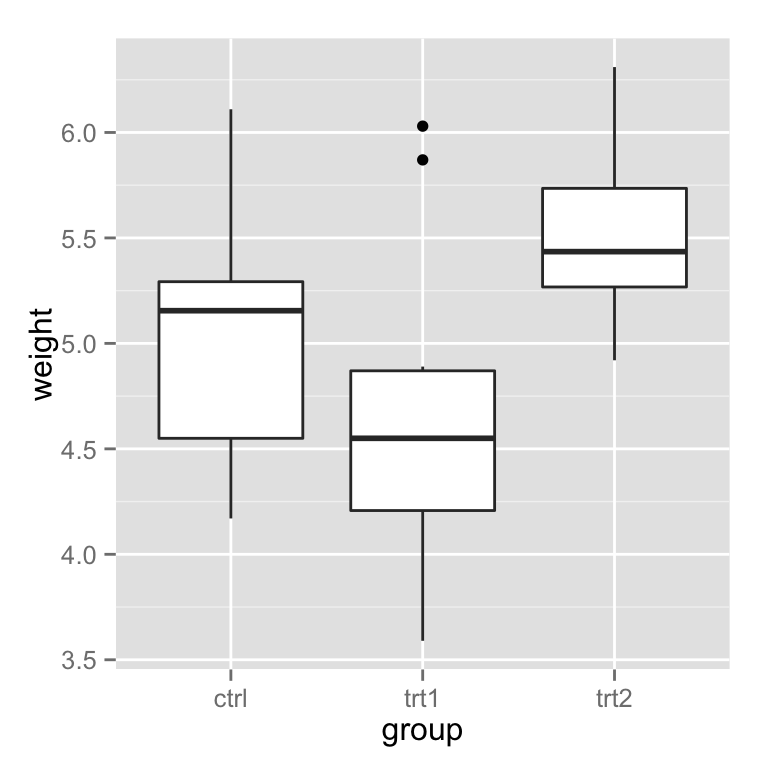反转 x轴 与 y轴
bp + coord_flip()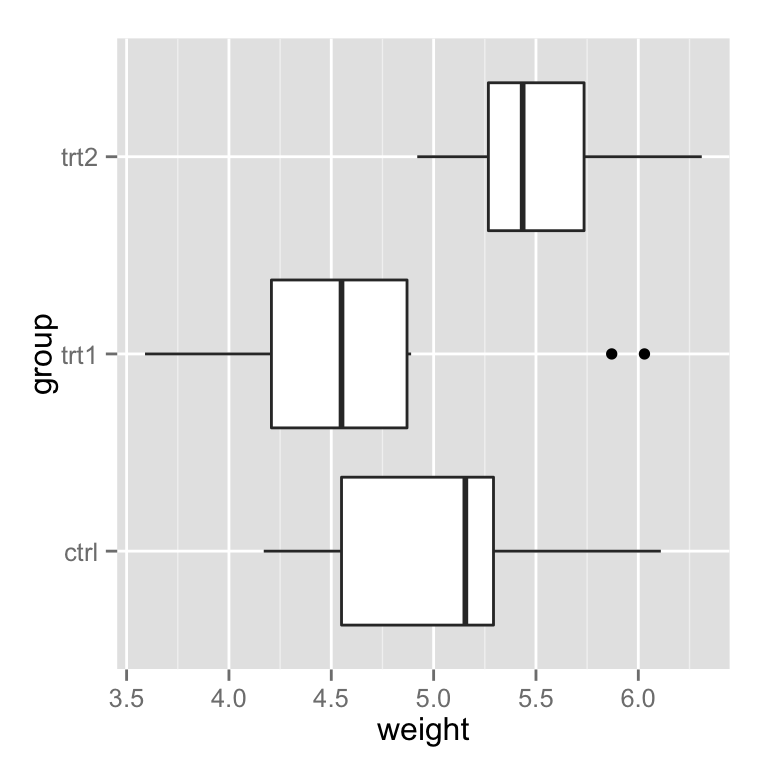离散型数据的坐标轴
改变坐标轴中各项目的顺序 > 特别注意, 离散数据的坐标轴中数据做为 factor 变量处理,他的位置取决于 level的顺序
# 手动设置x轴的位置
bp + scale_x_discrete(limits=c("trt1","trt2","ctrl"))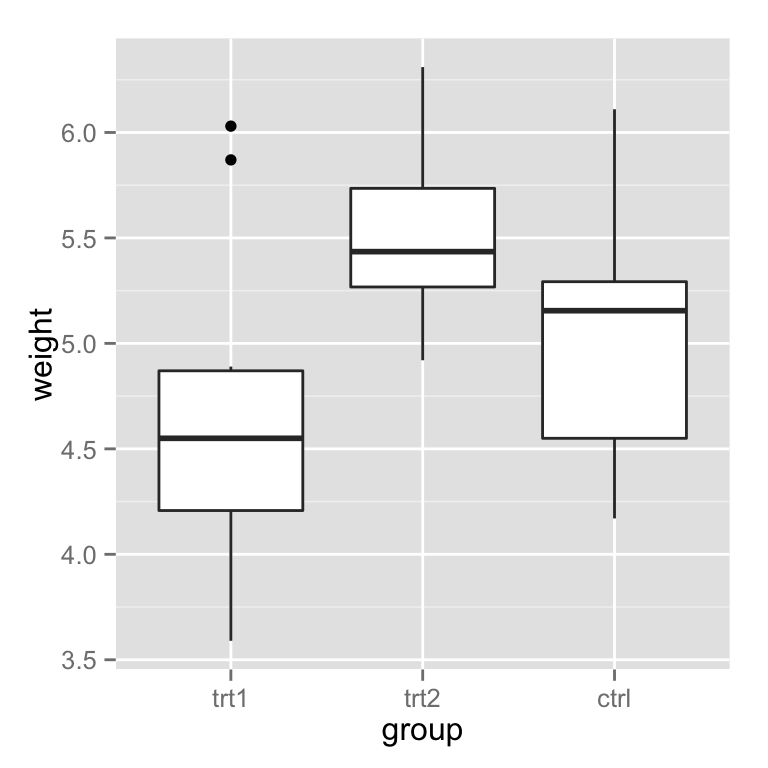# 逆转顺序
# 得到 factor 变量的 level
flevels <- levels(PlantGrowth$group) flevels ##  "ctrl" "trt1" "trt2" # 逆转了 level 的顺序 flevels <- rev(flevels) flevels ##  "trt2" "trt1" "ctrl" bp + scale_x_discrete(limits=flevels)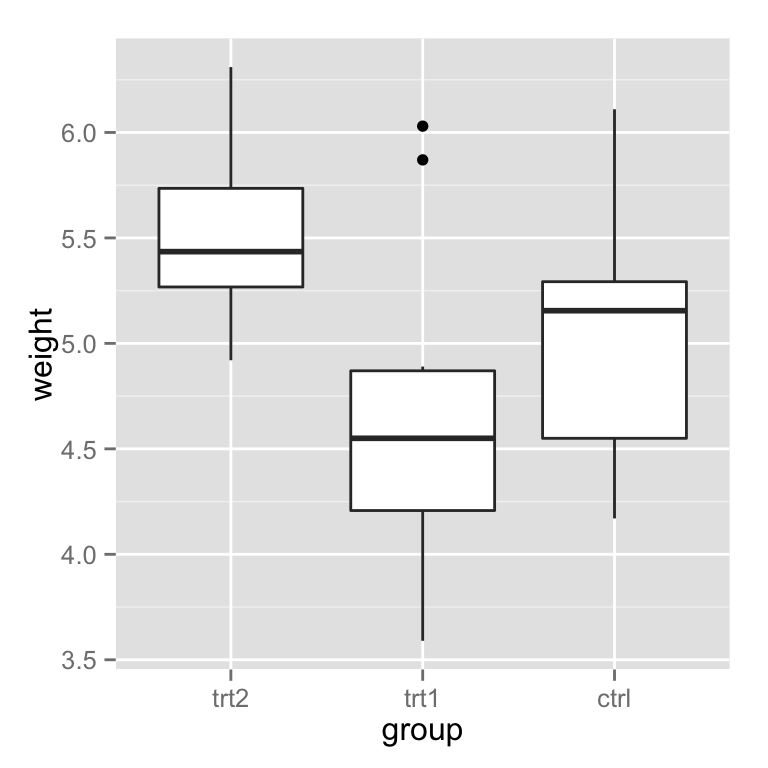# 或者写到一行里面 bp + scale_x_discrete(limits = rev(levels(PlantGrowth$group)))scale_x_discrete 可以设置离散型(discrete)数据, 中间的 x 表示处理x轴,如果是 fill 则可以修改填充颜色, color 修改边框颜色, shape 修改形状……

设置坐标轴的标签
# 将原有的 "ctrl", "trt1", "trt2" 修改为 "Control", "Treat 1", "Treat 2"
bp + scale_x_discrete(breaks=c("ctrl", "trt1", "trt2"),
labels=c("Control", "Treat 1", "Treat 2"))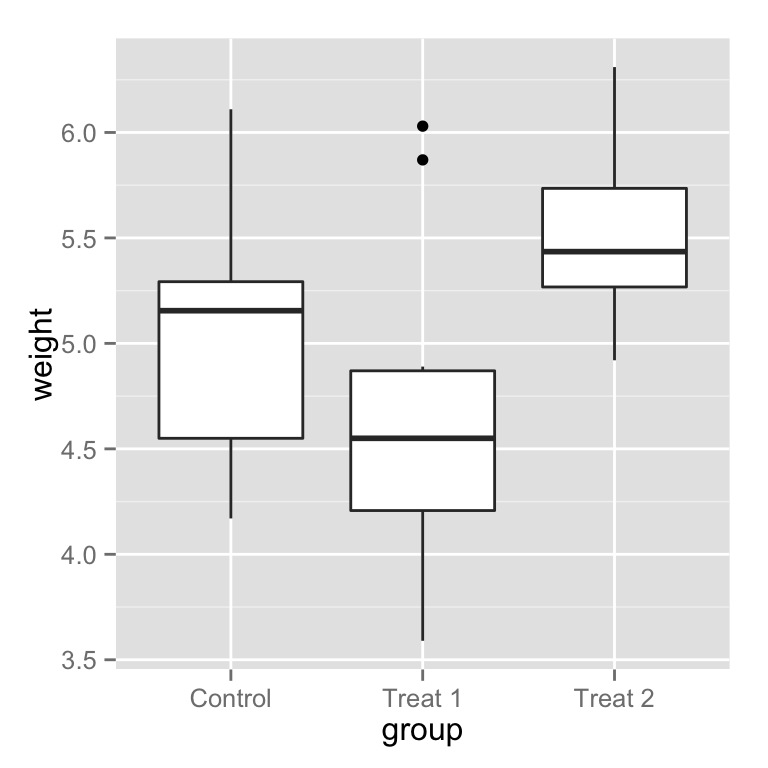# 隐藏
bp + scale_x_discrete(breaks=NULL)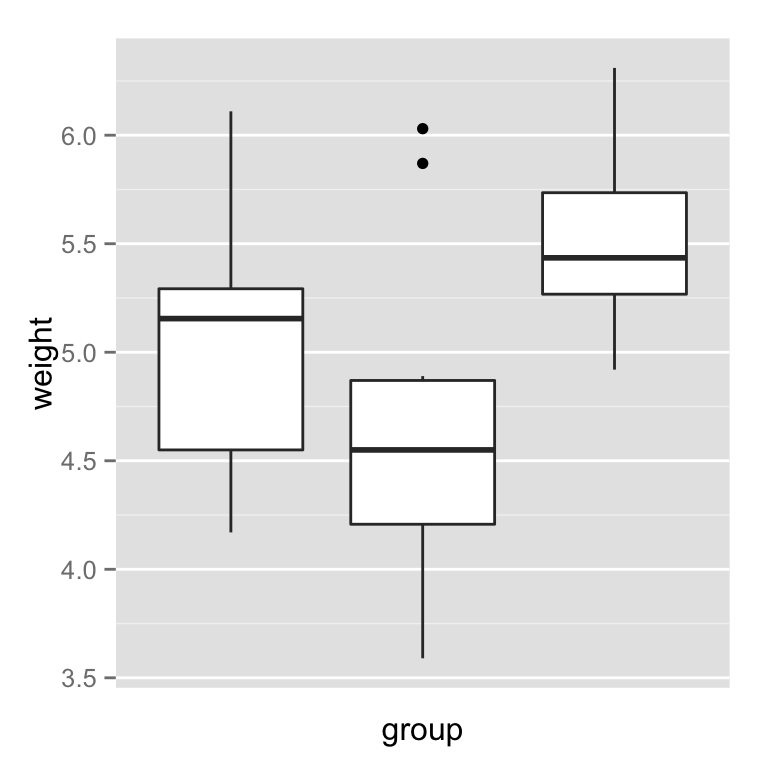# 也可以这样通过设置 theme 实现
bp + theme(axis.ticks = element_blank(), axis.text.x = element_blank())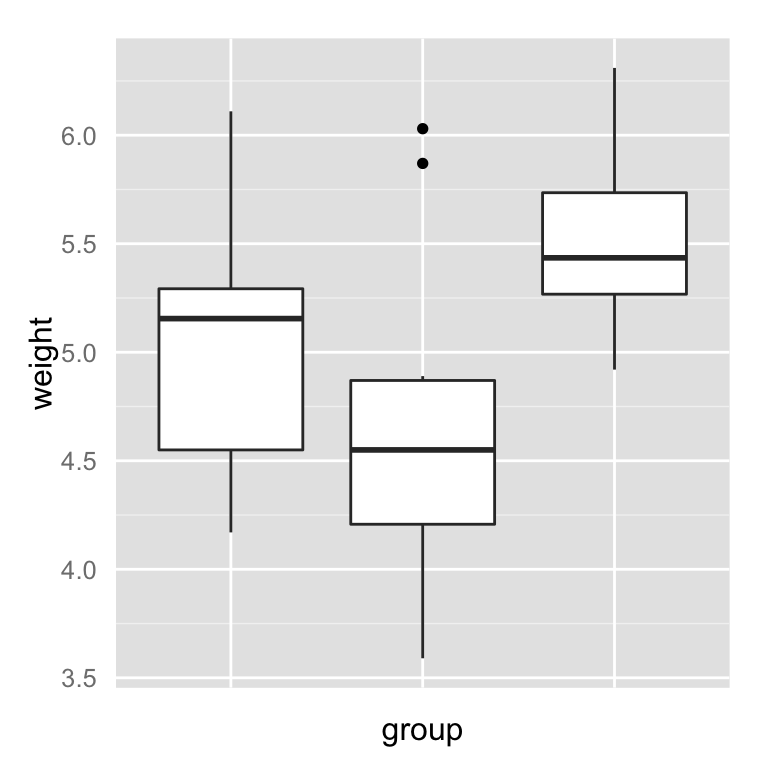连续型数据的坐标轴

设置坐标轴的范围和颠倒
# Make sure to include 0 in the y axis
bp + expand_limits(y=0) # y轴从0开始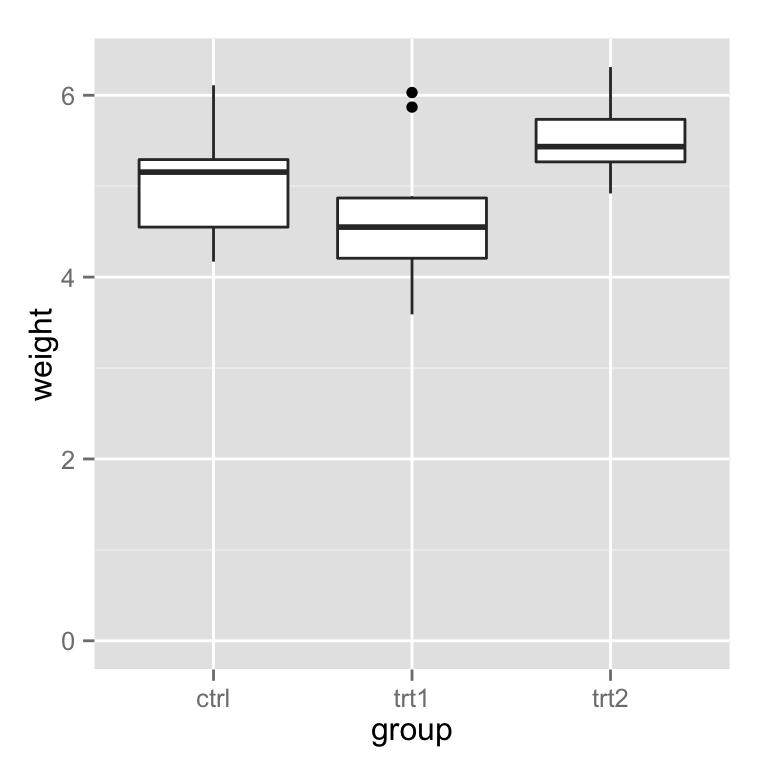# 设置y轴的范围
bp + expand_limits(y=c(0,8))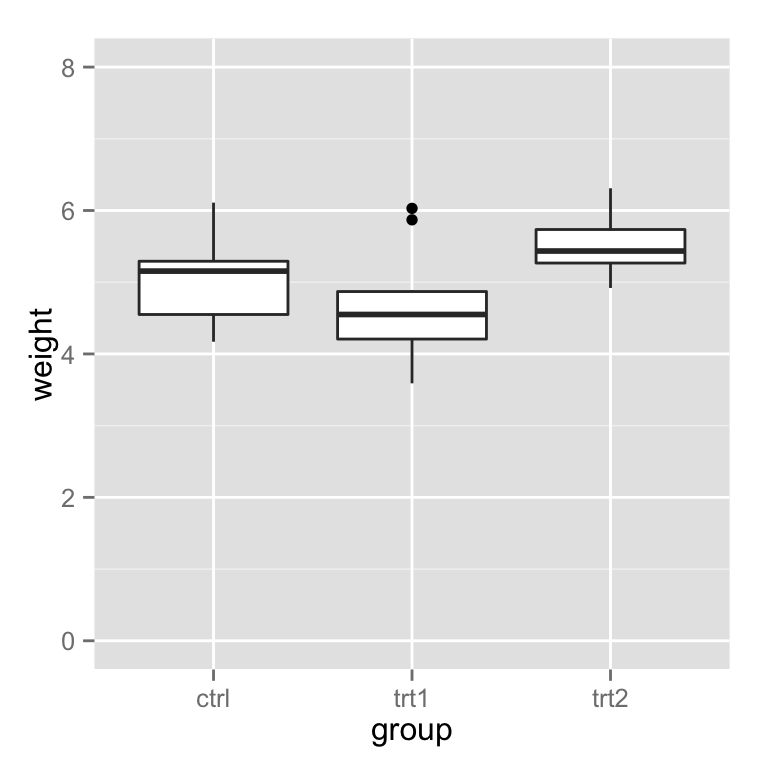我们可以通过expand_limits设置坐标轴的范围, 但是如果 scale_y_continuous 被使用, 那么就会覆盖ylim的设置.

# 设置y轴的范围
bp + ylim(0, 8)# 同样可以这样
bp + scale_y_continuous(limits=c(0, 8))# 如果同时设置 scale_y_continuous 和 ylim那么ylim会被覆盖,首先执行scale_y_continuous
bp + scale_y_continuous(limits=c(0, 8))+
ylim(0,10)
## Scale for 'y' is already present. Adding another scale for 'y', which will replace the existing scale.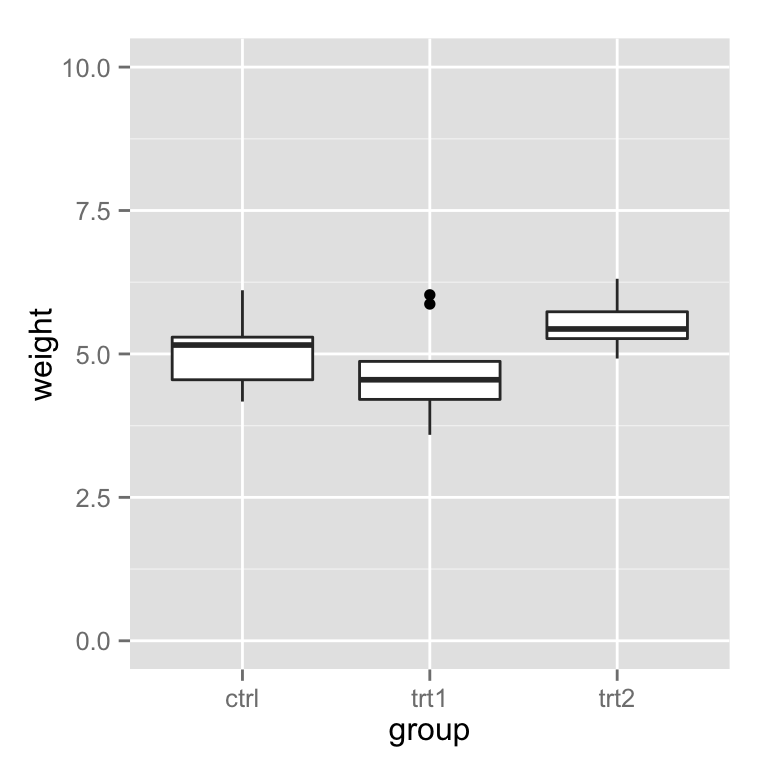如果 y 的范围使用上面的方法被截取, 那么这个范围以外的数据会被忽略,原始数据中的数据同样会被忽略,比如设置了ylim(5,8),那么小于5和大于8的原始数据同样会被忽略,当然散点图没有问题,但是箱线图会出错.
为了避免这个问题可以使用coord_cartesian来设置范围.
可以看下面的例子, 第一个出错了, 第二个使用了coord_cartesian得到了正确的绘图.
# These two do the same thing; all data points outside the graphing range are
# dropped, resulting in a misleading box plot
bp + ylim(5, 7.5)
## Warning: Removed 13 rows containing non-finite values (stat_boxplot).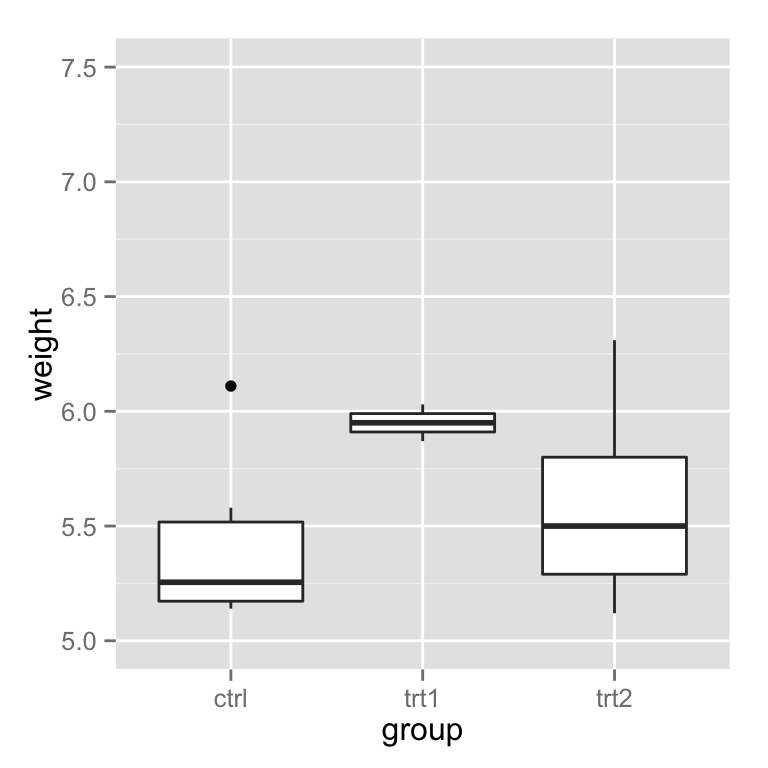# bp + scale_y_continuous(limits=c(5, 7.5))

# Using coord_cartesian "zooms" into the area
bp + coord_cartesian(ylim=c(5, 7.5))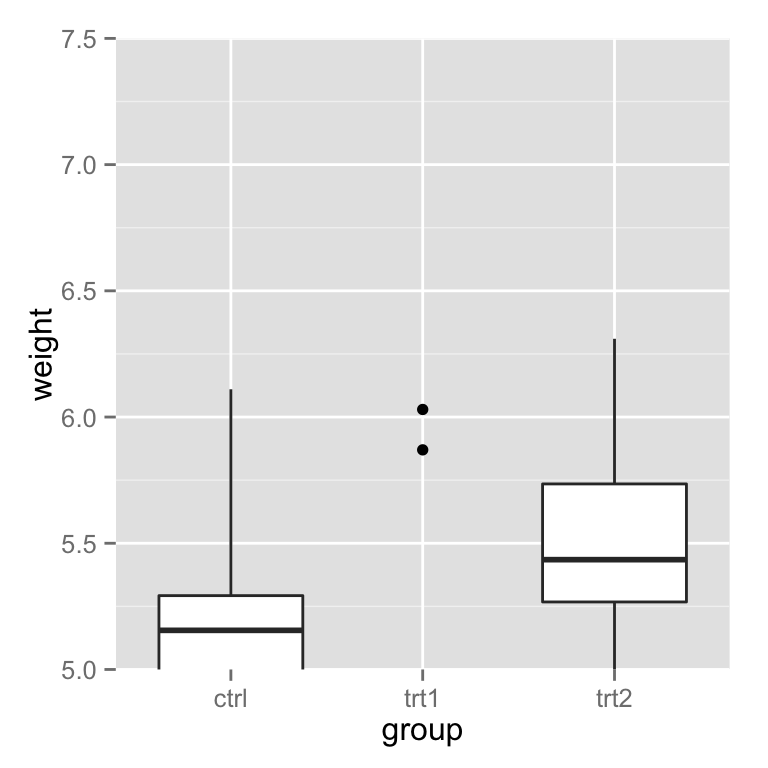# Specify tick marks directly
bp + coord_cartesian(ylim=c(5, 7.5)) +
scale_y_continuous(breaks=seq(0, 10, 0.25))  # Ticks from 0-10, every .25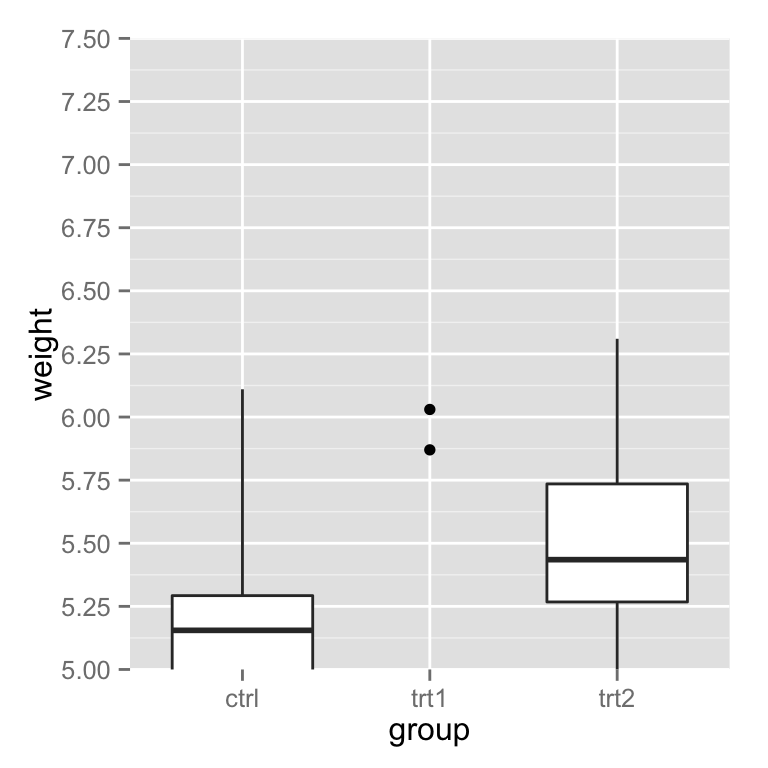### 点到坐标轴的方向
# Reverse order of a continuous-valued axis
bp + scale_y_reverse()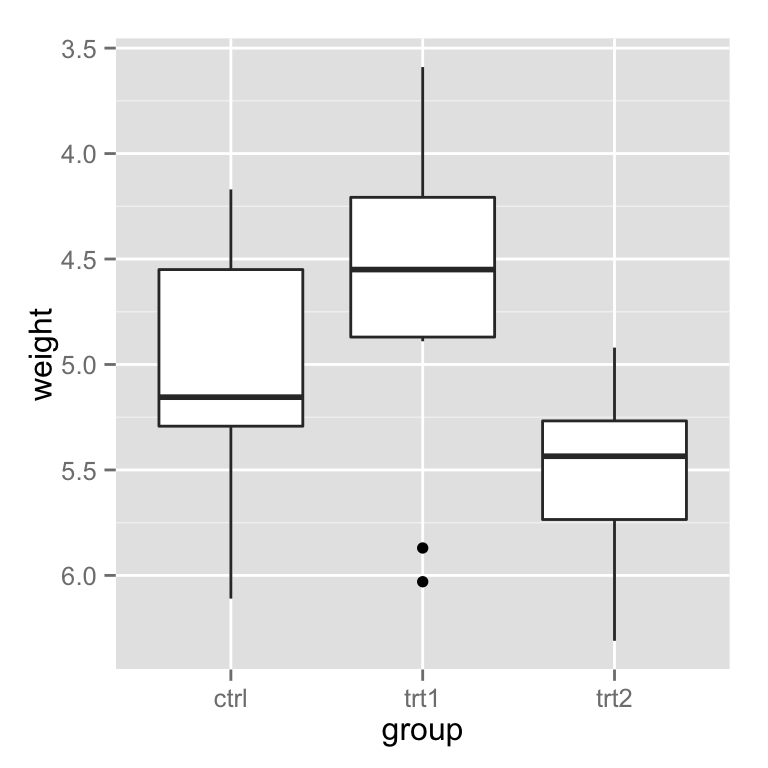设置和隐藏坐标轴的刻度
# Setting the tick marks on an axis
# 显示刻度从1到10,间隔为0.25
# The scale will show only the ones that are within range (3.50-6.25 in this case)
bp + scale_y_continuous(breaks=seq(1,10,1/4))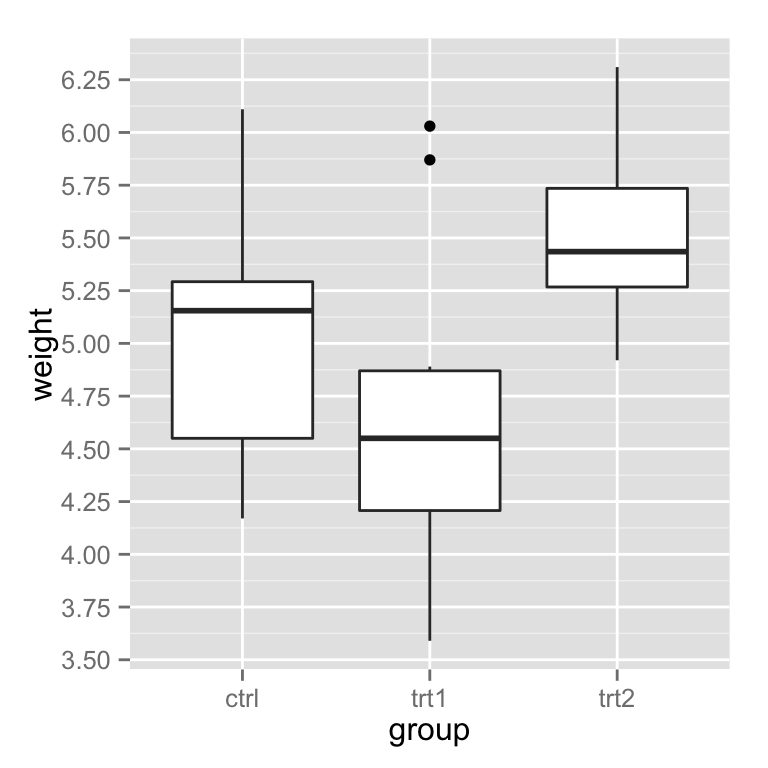# 未设置刻度的地方会出现空白
bp + scale_y_continuous(breaks=c(4, 4.25, 4.5, 5, 6,8))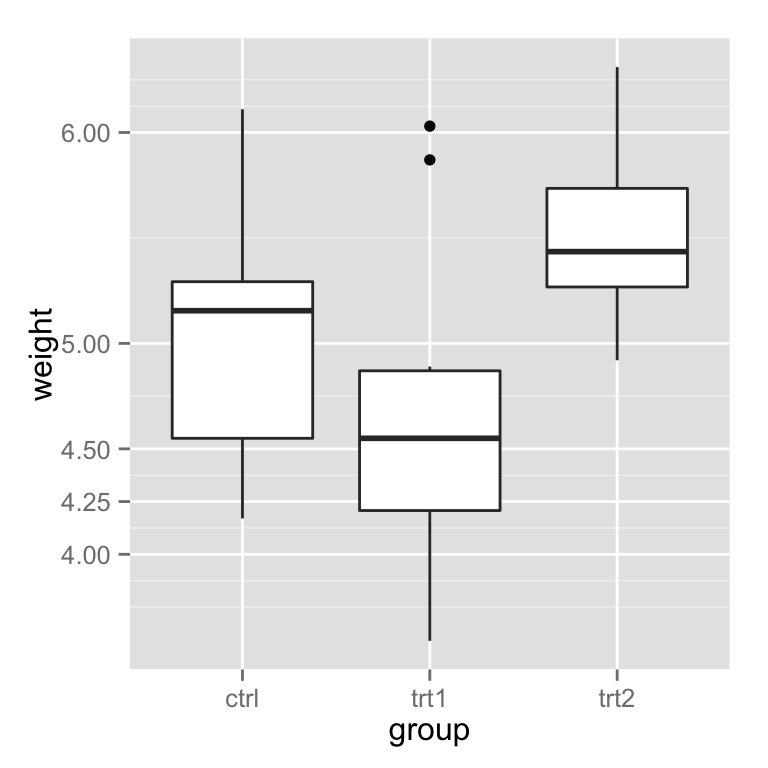# 隐藏刻度
bp + scale_y_continuous(breaks=NULL)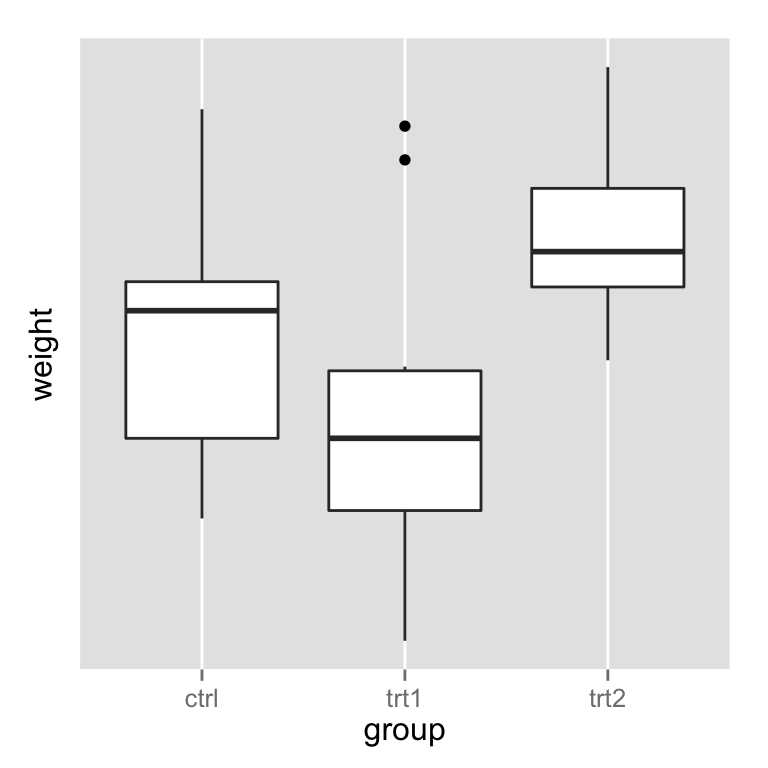# 隐藏刻度但是显示标签
bp + theme(axis.ticks = element_blank())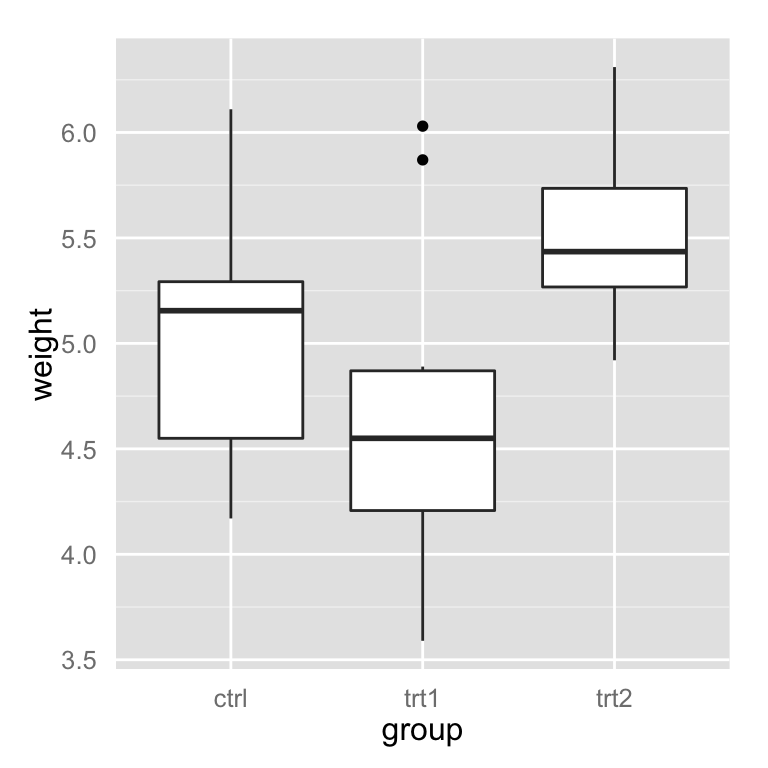坐标轴的数据转换(log, sqrt, etc.)
坐标轴可以进行线性变换,比如 log, power, roots 等等
这里有两种方式对数据进行转换, 一种是比例转换, 另一种是坐标转换. 对于比例变换, 在坐标轴刻度和范围被决定之前发生变换, 也就是先绘制图形,在标明刻度; 对于坐标变换, 在坐标轴刻度和范围被决定之后发生变换.也就是先标明刻度再绘制图形.
具体的理解可以看下面的例子.
# 指数分布的数据
set.seed(201)
n <- 100
dat <- data.frame(
xval = (1:n+rnorm(n,sd=5))/20,
yval = 2*2^((1:n+rnorm(n,sd=5))/20)
)

# 散点图
sp <- ggplot(dat, aes(xval, yval)) + geom_point()
sp